0

# 【C语言】指针和数组的深入理解（第三期）## 1、指针变量在口语中为什么会跟指针混用？

``````int main()
{
int a = 10; //当 a 做左值，a 是变量
int b = a; //当 a 做右值，a本质就是10

int* p1 = &a; //当 p1 做左值，p1是一个变量
int* p2 = p1; //当 p1 做右值 p1 等价于 &a，也就是a的地址，即指针
return 0;
}
``````

## 2.2 什么是数组指针？

int (p) 这样就是我们的数组指针，因为 [ ] 的优先级比 * 高，所以我们先 p 跟 * 结合，表示它是一个指针变量，指向的类型是 int，刚好跟我们 int p 的说法吻合。

## 2.3 指针数组和数组指针的布局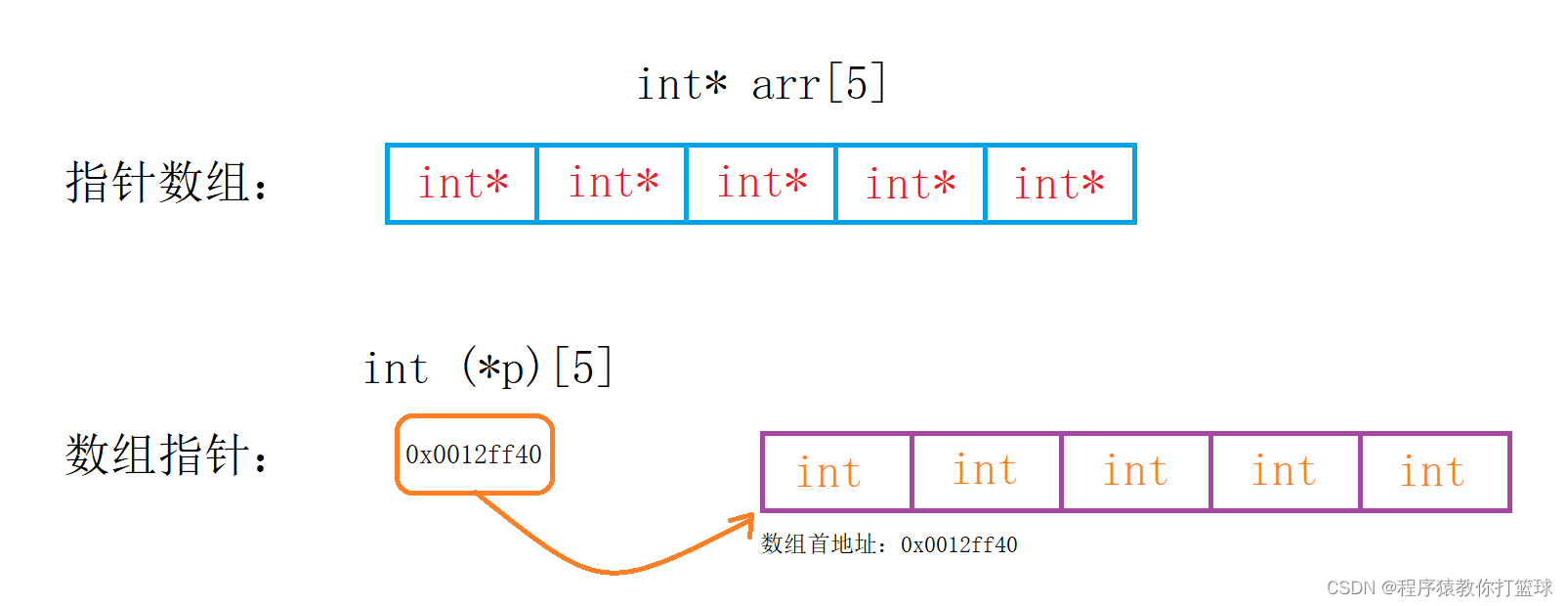## 2.4 数组指针的使用

``````void printArr1(int arr, int row, int col)
{
for (int i = 0; i < row; i++)
{
for (int j = 0; j < col; j++)
{
printf("%d ", arr[i][j]);
}
printf("\n");
}
}
void printAarr2(int(*arr), int row, int col)
{
for (int i = 0; i < row; i++)
{
for (int j = 0; j < col; j++)
{
printf("%d ", arr[i][j]);
}
printf("\n");
}
}
int main()
{
int arr = { 1, 2, 3, 4, 5, 6, 7, 8, 9, 10 };
printArr1(arr, 3, 5);
printAarr2(arr, 3, 5);
return 0;
}
``````

## 3.1 二维数组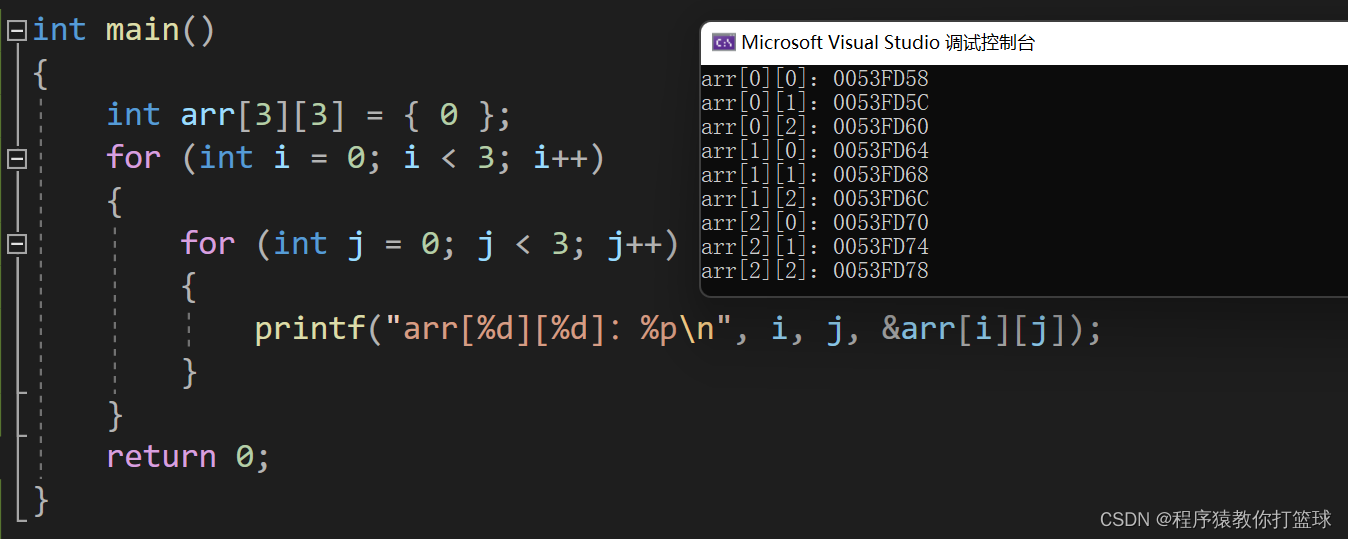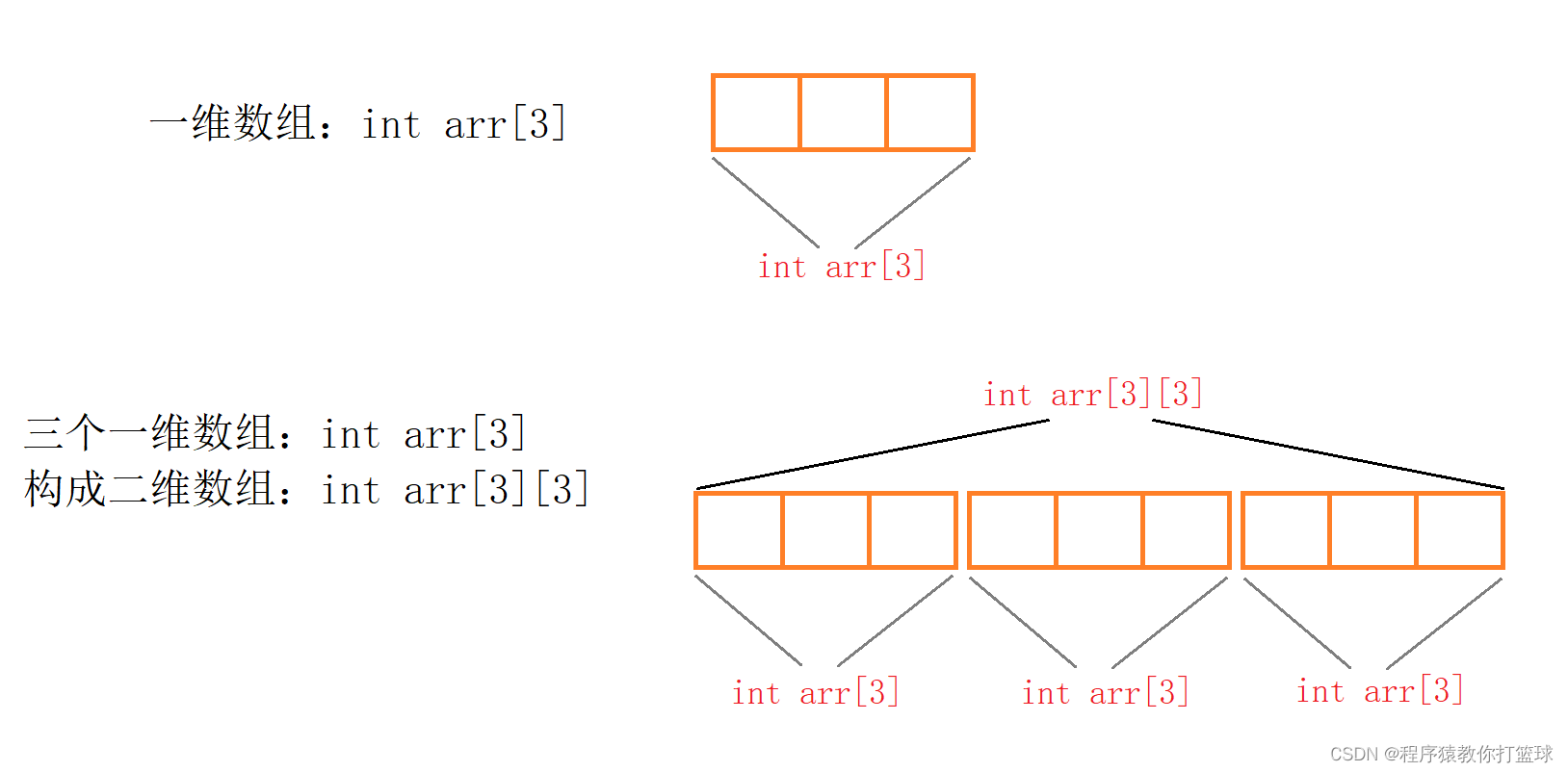``````int main()
{
int arr = { 0 };
int* p = (int*)arr;
for (int i = 0; i < 3 * 3; i++)
{
printf("%p\n", p + i);
}
return 0;
}
``````

``````int main()
{
int arr = { 0 };
printf("%d\n", sizeof(arr)); //36->计算的是整个数组的大小

printf("%d\n", sizeof(arr)); //4->算的是第一个元素的大小

printf("%d\n", sizeof(arr)); //12
//arr相当于第一行一维数组的数组名，单独放在sizeof内部
//所以算出的是第一行一位数组的大小

printf("%d\n", sizeof(arr + 1)); //4
//arr相当于第一行一维数组的数组名，数组名表示首元素地址，+1则跳过一个数组元素类型大小
//数组名没有单独放在sizeof内部，也没有取地址，所以求的是第一行第二个元素的大小

printf("%d\n", sizeof(*(arr + 1))); //4
//arr + 1相当于第二行数组名，对第二行数组名解引用，没有&，也没有单独放在sizeof内部
//所以求的是第二行第一个元素的大小->数组名代表首元素地址

printf("%d\n", sizeof(arr + 1)); //4或8
//arr表示数组首元素地址，+1表示跳过一个元素，所以求得是第二个元素地址的大小
//地址的大小在32位平台下为4字节，64位平台为8字节

printf("%d\n", sizeof(*(arr + 1))); //12
//数组名代表首元素地址，arr表示数组首元素，也就是第一行的一维数组
//arr+1就是第二行的一维数组的地址，对一维数组的地址解引用，访问的是整个数组，所以是12

printf("%d\n", sizeof(&arr + 1)); //4或8
//arr表示数组首元素，也就是第一行的一维数组，取地址取出的是一维数组的地址
//对数组+1本质跳过一个数组，也就表示第二行的地址，但它并没有单独出现在sizeof内部
//表示的是第二行首元素的地址，地址大小是4或8字节

printf("%d\n", sizeof(*(&arr + 1))); //12
//&arr + 1取出的是第二行一维数组的地址，对整个一维数组的地址解引用，访问的是整个数组

printf("%d\n", sizeof(*arr)); //12
//数组名代表首元素地址，解引用访问第一个元素，二维数组的第一个元素是第一行的整个一维数组
//所以*arr也就是第一行的数组名即arr，单独放在了sizeof内部，计算的是整个一维数组的大小

printf("%d\n", sizeof(arr)); //12
//arr表示二维数组的第二个元素也就是第二行的数组名，同上，计算的是整个一维数组的大小

return 0;
}
``````

## 3.2 二级指针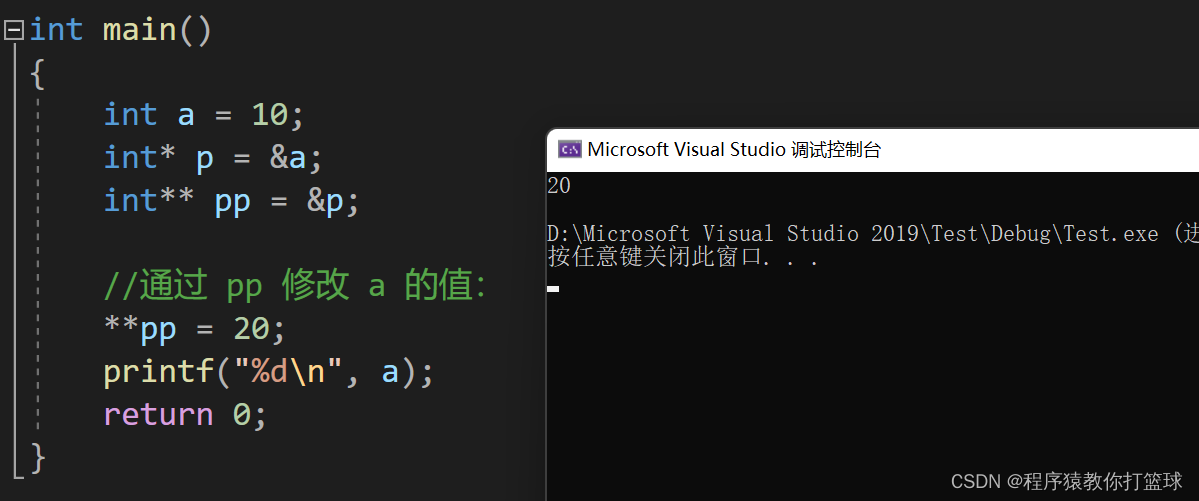## 3.3 一道面试题

``````int main()
{
int arr;
int(*p);
p = arr;
printf("%p,%d\n", &p - &arr, &p - &arr);
return 0;
}
``````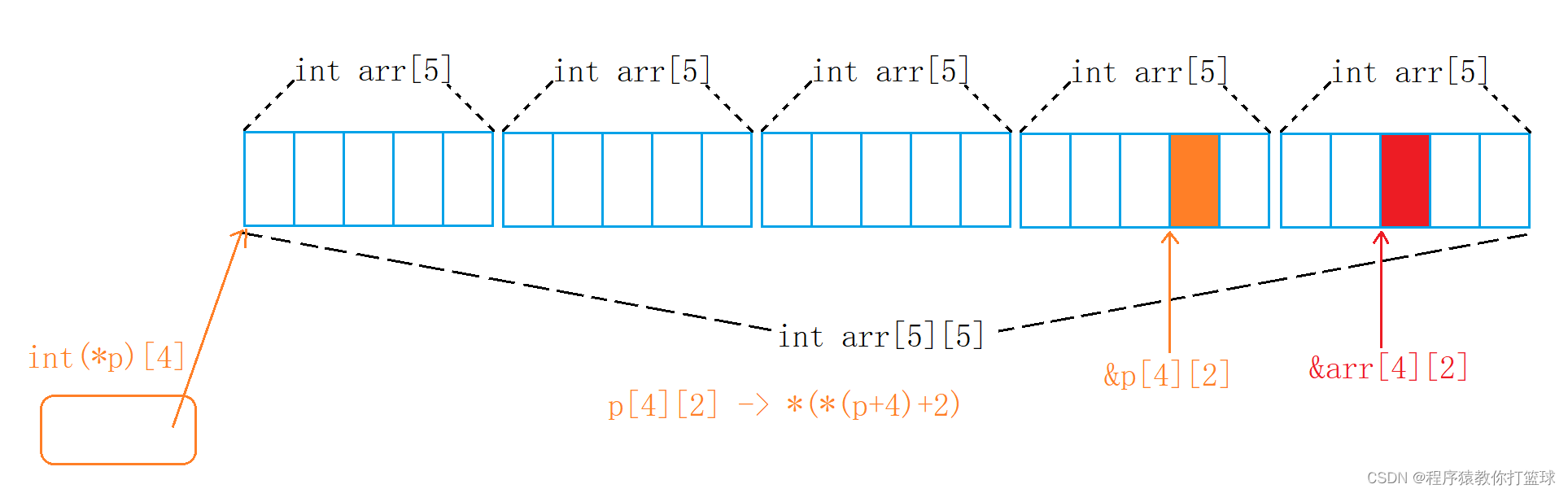### “【C语言】指针和数组的深入理解（第三期）”的评论:

##### 关于作者##### overfit同步小助手